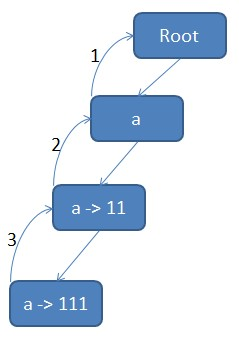# 基于 AC 自动机的多模匹配的 map

```#include <iostream>
#include <stdio.h>
#include <memory.h>
#include <string>
#include <queue>
#include <set>

#define CHAR_COUNT 128

class Node {
public:
Node() {
memset(this, 0, sizeof(*this));
}
char ch;
Node* fail;
Node* next[CHAR_COUNT];
bool end;
int value;
};

class Trie {
public:
Node* root;

Trie() {
root = new Node();
}

~Trie() {
clear(root);
}

void insert(const char* strKey, int value) {
Node* p = root;
for (int i = 0; strKey[i]; i++) {
char ch = strKey[i];
if (p->next[ch] == NULL) {
p->next[ch] = new Node();
}
p = p->next[ch];
p->ch = strKey[i];
}
p->end = true;
p->value = value;
}

void build() {
std::queue<Node*> q;
q.push(root);
while ( ! q.empty()) {
Node* tmp = q.front();
q.pop();
for (int i = 0; i < CHAR_COUNT; i++) {
if (tmp->next[i]) {
if (tmp == root) {
tmp->next[i]->fail = root;
} else {
Node* p = tmp->fail;
while (p) {
if (p->next[i]) {
tmp->next[i]->fail = p->next[i];
break;
}
p = p->fail;
}
if ( ! p) {
tmp->next[i]->fail = root;
}
}
q.push(tmp->next[i]);
}
}
}
}

std::vector<int> query(const char* str) {
std::vector<int> result;
Node* p = root;
for (int i = 0; str[i]; i++) {
char ch = str[i];
while ( ! p->next[ch] && p != root) {
p = p->fail;
}
p = p->next[ch];
if ( ! p) {
p = root;
}
Node* tmp = p;
while (tmp != root) {
if (tmp->end) {
result.push_back(tmp->value);
}
tmp = tmp->fail;
}
}
return result;
}

void clear(Node*& p) {
if (p) {
for (int i = 0; i < CHAR_COUNT; i++) {
clear(p->next[i]);
}
delete p;
p = NULL;
}
}
};

int main() {
int t;
scanf("%d", &t);
while (t--) {
Trie trie;
int n;
scanf("%d", &n);
while (n--) {
std::string keyword;
int value;
std::cin>>keyword>>value;
trie.insert(keyword.c_str(), value);
}
trie.build();
std::string query;
std::cin>>query;
std::vector<int> result = trie.query(query.c_str());
printf("size %d\n", result.size());
for (std::vector<int>::iterator it = result.begin(); it != result.end(); it++) {
printf("%d\n", *it);
}
}
return 0;
}
```

```1
2
11
11
111
111
111
```

```size 3
11
111
11
```1. 第 50 行，把 root 节点加入队列（root 节点的失败指针是 NULL），然后进入 51 行开始的队列处理循环
2. 取出 root 节点，根据 56 – 58 行，对 root 节点的所有直接子节点，将其失败指针指向 root，对应图上的失败指针 1。同时 71 行将 root 节点的所有子节点追加入队列。至此，队列中有一个节点，也就是第一个 a 节点。
3. 回到 52 行，取出第一个 a 节点，开始处理。注意到在 54 – 73 行的 for 循环中，tmp 指针是一直不会发生变化的，所以，这个 tmp 指针，可以理解成“当前父节点”，而在此轮循环中，任务就是为这个“当前父节点”的全部非空子节点找好失败指针。那么，对于第一个 a 节点，代码走到 59 行，指针 p 指向第一个 a 节点的失败指针，也就是 root，然后进入 60 – 66 的 while 循环。由于 root 节点有一个字符为 a 的子节点，于是在第一轮的循环中，就为第二个 a 节点找好了失败指针，也就是让第二个 a 节点的失败指针指向第一个 a 节点。
4. 第二个 a 节点成为“当前父节点”，同理，为其子节点（也就是第三个 a 节点）找到的失败指针指向了第二个 a 节点。至此所有失败指针建立完成。

1. 对于第一个字母，走到第一个 a 节点，沿着失败指针找，找到 root，跳出
2. 对于第二个字母，走到第二个 a 节点，然后他的 end 标志是 true 的，于是把这个节点的 value 值 11 加入结果集，沿着失败指针，找到第一个 a 节点，再沿着失败指针，找到 root 节点，跳出
3. 对于第三个字母，走到第三个 a 节点，并发现第三个 a 节点的 end 标志是 true，于是把这个节点的 value 值 111 加入结果集，并继续沿着失败指针，找到第二个 a 节点，发现他是 end 的，于是将 11 加入结果集，再沿着失败指针，找到第一个 a 节点和 root 节点，跳出。

```#include <iostream>
#include <stdio.h>
#include <memory.h>
#include <string>
#include <queue>
#include <map>
#include <set>

#define CHAR_COUNT 128

class Node {
public:
Node() {
ch = 0;
fail = NULL;
memset(next, NULL, sizeof(next));
end = false;
key = "";
value = 0;
}
char ch;
Node* fail;
Node* next[CHAR_COUNT];
bool end;
std::string key;
int value;
};

class Trie {
public:
Node* root;

Trie() {
root = new Node();
}

~Trie() {
clear(root);
}

void insert(const char* strKey, int value) {
Node* p = root;
for (int i = 0; strKey[i]; i++) {
char ch = strKey[i];
if (p->next[ch] == NULL) {
p->next[ch] = new Node();
}
p = p->next[ch];
p->ch = strKey[i];
}
p->end = true;
p->key.assign(strKey);
p->value = value;
}

void build() {
std::queue<Node*> q;
q.push(root);
while ( ! q.empty()) {
Node* tmp = q.front();
q.pop();
for (int i = 0; i < CHAR_COUNT; i++) {
if (tmp->next[i]) {
if (tmp == root) {
tmp->next[i]->fail = root;
} else {
Node* p = tmp->fail;
while (p) {
if (p->next[i]) {
tmp->next[i]->fail = p->next[i];
break;
}
p = p->fail;
}
if ( ! p) {
tmp->next[i]->fail = root;
}
}
q.push(tmp->next[i]);
}
}
}
}

std::map<std::string, int> query(const char* str) {
std::map<std::string, int> result;
Node* p = root;
for (int i = 0; str[i]; i++) {
char ch = str[i];
while ( ! p->next[ch] && p != root) {
p = p->fail;
}
p = p->next[ch];
if ( ! p) {
p = root;
}
Node* tmp = p;
while (tmp != root) {
if (tmp->end) {
result[tmp->key] = tmp->value;
}
tmp = tmp->fail;
}
}
return result;
}

void clear(Node*& p) {
if (p) {
for (int i = 0; i < CHAR_COUNT; i++) {
clear(p->next[i]);
}
delete p;
p = NULL;
}
}
};

int main() {
int t;
scanf("%d", &t);
while (t--) {
Trie trie;
int n;
scanf("%d", &n);
while (n--) {
std::string keyword;
int value;
std::cin>>keyword>>value;
trie.insert(keyword.c_str(), value);
}
trie.build();
std::string query;
std::cin>>query;
std::map<std::string, int> result = trie.query(query.c_str());
printf("size %d\n", result.size());
for (std::map<std::string, int>::iterator it = result.begin(); it != result.end(); it++) {
printf("%s -> %d\n", it->first.c_str(), it->second);
}
}
return 0;
}
```

## 4 thoughts on “基于 AC 自动机的多模匹配的 map”

1.wanax on said:

虽不明但觉厉…

•ZRJ on said:

其实这个还是很有应用价值的，例如说，发微博，或者留言的时候，需要敏感词过滤，敏感词可能有成千上万个，一条留言大约两三百个字符，你总不能循环的调用成千上万次的 str find 函数吧，这个时候就要靠这个 ac 自动机了

•wanax on said:

这个就是那个论文的东西？

•ZRJ on said:

啥论文。。怎么会扯到论文去了